# Substantial Maths Lesson Starters

If you are looking for a Maths lesson starter that can develop into a whole lesson then try the list on the left side of this page.

The starters here have been selected because they can develop into projects, investigations and activities that require more time than the first minutes of a lesson. The optionsl activities also provide a lot more for the student to do online.

Main Page

### Substantial Starters: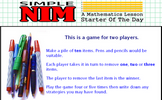Simple Nim: The classic game of Nim played with a group of pens and pencils. The game can be extended to the multi-pile version. An optional student activity is linked from the bottom of this starter page called Nim. Nim is a mathematical game of strategy in which two players take turns removing objects from groups of objects..Missing Square Puzzle: The missing square puzzle is an optical illusion used to help students reason about geometrical figures. An optional student activity is linked from the bottom of this starter page called Area of a Triangle. Calculate the areas of the given triangles in this self marking quiz..Roman Numerals: Learn a very different method for writing numbers using Roman numerals. An optional student activity is linked from the bottom of this starter page called Roman Numerals Jigsaw. An online interactive jigsaw puzzle of a grid of Roman numerals..Rail Weigh: Use the weights of the trains to work out the weight of a locomotive and a coach. A real situation which produces simultaneous equations. An optional student activity is linked from the bottom of this starter page called Simultaneous Shopping. Find the cost of the individual items in shopping baskets (introduction to simultaneous equations)..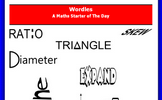Wordles: Turn mathematical words into designs which will help you remember the meanings of the words. An optional student activity is linked from the bottom of this starter page called Shark's Dinner. The mathematical version of the classic hangman game. Guess the letters that are contained in the words..Coins in Envelopes: Fifteen pennies are placed in four envelopes and the envelopes are sealed. It is possible to pay someone any amount from 1p to 15p by giving them one or more envelopes. An optional student activity is linked from the bottom of this starter page called Stamp Sticking. Drag stamps onto the envelopes to make the exact postage as shown at the top left of each envelope..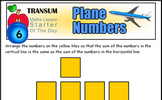Plane Numbers: Arrange numbers on the plane shaped grid to produce the given totals An optional student activity is linked from the bottom of this starter page called Plane Numbers. Arrange numbers on the plane shaped grid to produce the given totals.Snowman and Woman: How many different ways can you spell out the word snowman by moving from snowflake to snowflake. An optional student activity is linked from the bottom of this starter page called Xmas Tree Trim. Use the lights provided to decorate each of the Christmas trees so that no two trees are the same..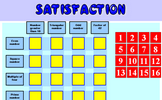Satisfaction: Rearrange the numbers, row and column headings so that this table is mathematically correct. An optional student activity is linked from the bottom of this starter page called Satisfaction. This is quite a challenging number grouping puzzle requiring a knowledge of prime, square and triangular numbers..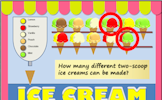Ice Cream: How many different ice cream cones can be made by choosing two scoops from six flavours? An optional student activity is linked from the bottom of this starter page called Striped Sweets. Learn how to be systematic by colouring the sweet wrappers so that no two are the same..Noughts and Crosses: In how many different ways can the first X and O by placed on the grid? An optional student activity is linked from the bottom of this starter page called Transum Club. How many different colour schemes can you devise for the Transum Club Badge..Handshakes: If all the students in this room shook hands with each other, how many handshakes would there be altogether? An optional student activity is linked from the bottom of this starter page called Mystic Rose. Investigate the properties of the Mystic Rose by using this interactive diagram..THOAN: THOAN stands for 'Think of a number' and there are four randomly generated THOAN puzzles to solve. An optional student activity is linked from the bottom of this starter page called Think of a Number. Ten students think of a number then perform various operations on that number. You have to find what the original numbers were..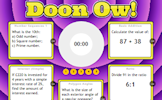Consecutive Squares: What do you notice about the difference between the squares of consecutive numbers? An optional student activity is linked from the bottom of this starter page called Square Pairs Game. A game for two players who take turns to select two numbers that add up to a square number..Red Lines: Either estimate the lengths of the red lines or, if you know how, calculate how long they are. An optional student activity is linked from the bottom of this starter page called Pythagoras. An online exercise to test your understanding of and ability to apply Pythagoras' Theorem..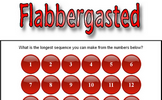Flabbergasted: If each number in a sequence must be a factor or multiple of the previous number what is the longest sequence that can be made from the given numbers? An optional student activity is linked from the bottom of this starter page called Factor Trees. Create factor trees to find the prime factors of the given numbers..Pears Make Squares: Find three numbers such that each pair of numbers adds up to a square number. An optional student activity is linked from the bottom of this starter page called Square and Even. Arrange the numbers on the cards so that each of the three digit numbers formed horizontally are square numbers and each of the three digit numbers formed vertically are even..Wrong Way Round: Find calculations which written back to front give the same answer. An optional student activity is linked from the bottom of this starter page called Palindromic numbers. How many steps does each number take to become palandromic?.Summer Holidays: How many children and how many donkeys are on the beach? You can work it out from the number of heads and the number of feet! An optional student activity is linked from the bottom of this starter page called Unbeknownst. Some picture grid puzzles which can be solved by using simultaneous equations..Two Twins and Tim: A tricky problem set on a coordinate grid. An optional student activity is linked from the bottom of this starter page called Coordinates. A self marking exercise on identifying coordinates culminating in finding the mid point of two given points..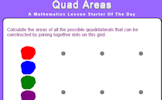Quad Areas: Calculate the areas of all the possible quadrilaterals that can be constructed by joining together dots on this grid. An optional student activity is linked from the bottom of this starter page called Pin Board. Rows and columns of dots that can be joined using straight lines to create shapes..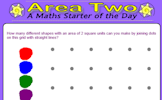Area Two: How many different shapes with an area of 2 square units can you make by joining dots on this grid with straight lines? An optional student activity is linked from the bottom of this starter page called Area and Perimeter. Show that you know the area and perimeter formulas of basic shapes..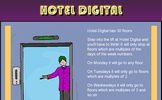Hotel Digital: A puzzle about the lifts in a hotel which serve floors based on the day of the week. An optional student activity is linked from the bottom of this starter page called Cryptographic. Fill in the squares according to the clues given by the string of numbers for each row and column..Verruca Value: The Verruca Value of a word is the number of vowels multiplied by the number of consonants. How many words can you find with Verruca Value of 24? An optional student activity is linked from the bottom of this starter page called Mental Strategies. Practise your mental arithmetic skills and learn some new strategies with this self marking exercise..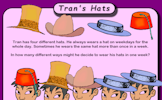Tran's Hats: In how many different ways might Tran decide to wear his hats in one week? An optional student activity is linked from the bottom of this starter page called Ice-cream Combinations. How many two-scoop ice creams can you make from the given flavours?.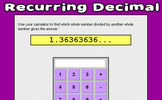Recurring Decimal: Use your calculator to find which whole number divided by another whole number gives a recurring decimal. An optional student activity is linked from the bottom of this starter page called Recurring Decimals. Change recurring decimals into their corresponding fractions and vica versa..Justundera Quid: Find a word whose letters would cost exactly ninety nine pence. An optional student activity is linked from the bottom of this starter page called Letter Sums. Use your mental arithmetic skills to add up the values of the letters in these mathematical words..Four's Independence: A clock face containing only the number 4. Can you make a clock face containing any other single number? An optional student activity is linked from the bottom of this starter page called One Digit Only. Find expressions using only one digit which equal the given targets..Weather Report: Find five different integers that multiply together to give a product of twelve. An optional student activity is linked from the bottom of this starter page called . .Simultaneous Occasions: A problem which can best be solved as a pair of simultaneous equations. An optional student activity is linked from the bottom of this starter page called Simultaneous Solutions. Arrange the given pairs of simultaneous equations in groups to show whether they have no solution, one solution or infinite solutions..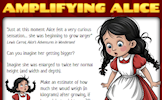Big Bieber: If the dimensions of an object double, its volume increases by a factor of eight. An optional student activity is linked from the bottom of this starter page called Similar Shapes. Questions about the scale factors of lengths, areas and volumes of similar shapes..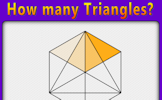How Many Triangles? 3: Find a systematic way of counting the number of triangles in the given diagram. An optional student activity is linked from the bottom of this starter page called How Many Squares? 2. How many different sets of four dots can be joined to form a square?.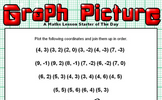Graph Picture: Plot the given coordinates then join them up to form a picture. An optional student activity is linked from the bottom of this starter page called Coordinates Picture. Plot the coordinates and join them with straight lines to produce a picture..Bizarre Triangle: By how much would the area of this triangle increase if its base was enlarged to 8cm? An optional student activity is linked from the bottom of this starter page called Pythagoras Basics. A drill and practice self marking exercise on the basics of Pythagoras' theorem..Less Than: This mathematics lesson starter invites pupils to interpret a three part algebraic inequality. An optional student activity is linked from the bottom of this starter page called Inequalities. Check that you know what inequality signs mean and how they are used to compare two quantities. Includes negative numbers, decimals, fractions and metric measures..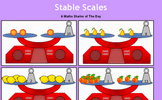Stable Scales: Solve these balance puzzles by taking the same away from both sides. An introduction to linear equations. An optional student activity is linked from the bottom of this starter page called Stable Scales. Ten balance puzzles to prepare you for solving equations..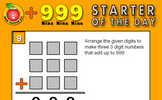Nine Nine Nine: Arrange the numbers 1-9 to make three 3 digit numbers that add up to 999. An optional student activity is linked from the bottom of this starter page called Nine Nine Nine. Use the digits 1 to 9 to make three 3 digit numbers which add up to 999..

### Other

Is there anything you would have a regular use for that we don't feature here? Please let us know.

Contact Transum

##### Recently Updated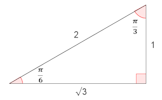A self-marking exercise on finding the exact values of sine, cosine and tangent of special angles given in radians. So far this activity has been accessed 72 times and 2 Transum Trophies have been awarded for completing it.

### Feedback

Do you have any comments? It is always useful to receive feedback and helps make this free resource even more useful for those learning Mathematics anywhere in the world. Click here to enter your comments.For All: